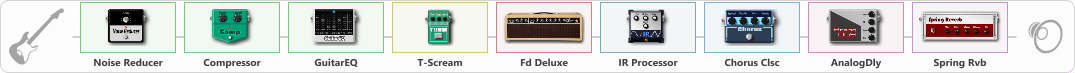# SRV strat bluesw

Discussion in 'ToneLib-GFX presets' started by truss van halen, Aug 4, 2020.

1. ### truss van halenWell-Known Member

SRV strat bluesw

Preset name: SRV Blues strat

Effects chain:Effect: "Noise Reducer" (Dynamics / Filter), active - "yes"
{
"Sens" = 100
"Mode" = Hard
}

Effect: "Compressor" (Dynamics / Filter), active - "yes"
{
"Sense" = 23
"Level" = 36
}

Effect: "GuitarEQ" (Dynamics / Filter), active - "yes"
{
"160 Hz" = 0
"400 Hz" = 1
"800 Hz" = -3
"1.6 kHz" = -6
"3.2 kHz" = 3
"6.4 kHz" = 2
"12 kHz" = -6
"Level (dB)" = 0
}

Effect: "T-Scream" (Overdrive / Distortion), active - "yes"
{
"Drive" = 16
"Tone" = 68
"Level" = 47
}

Effect: "Fd Deluxe" (Amp simulators), active - "yes"
{
"Gain" = 48
"Bass" = 41
"Middle" = 60
"Treble" = 66
"Presence" = 64
"Master" = 100
"Level (dB)" = 5
}

Effect: "IR Processor" (Cabinets), active - "yes"
{
"IR" = Fender Champ 57 On Axis, 1 in
"Low Cut (Hz)" = 44
"Hi Cut (kHz)" = 20.0
"Mix" = 100
"Level (dB)" = 0
}

Effect: "Chorus Clsc" (Modulation / Sfx), active - "yes"
{
"Speed" = 2.4
"Depth" = 33
"Center" = 2.6
"Mode" = Vibrato
}

Effect: "AnalogDly" (Delay), active - "yes"
{
"Time" = 596
"Feedback" = 28
"Tone" = 56
"Mix" = 35
}

Effect: "Spring Rvb" (Reverb), active - "yes"
{
"Time" = 4.9
"PreDelay" = 0
"LoDamp" = 16
"HiDamp" = 26
"Mix" = 37
}

Note: You will need to download and install the ToneLib-GFX software to use the preset.

#### Attached Files:

• ###### SRV_strat_bluesw.tlgfx
File size:
4.6 KB
Views:
4,187
2. супер